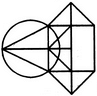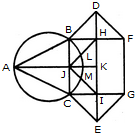# Non Verbal Reasoning - Analytical Reasoning - Discussion

Discussion Forum : Analytical Reasoning - Section 1 (Q.No. 15)
15.

Find the number of triangles in the given figure.10
12
14
16
Explanation:

The figure may be labelled as shown.The simplest triangles are ABJ, ACJ, BDH, DHF, CIE and GIE i.e. 6 in number.

The triangles composed of two components each are ABC, BDF, CEG, BHJ, JHK, JKI and CJI i.e. 7 in number.

There is only one triangle JHI which is composed of four components.

Thus, there are 6 + 7 + 1 = 14 triangles in the given figure.

Discussion:
3 comments Page 1 of 1.

Gavey said:   2 years ago